Wednesday, April 13, 2011

Information

The book features Claude Shannon prominently. It's unusual, including long discussions of (among other topics): African drum language, Charles Babbage, Shannon's information, Turing, quantum computing, and Wikipedia. It's broader and not as technical as I'd hoped, but very enjoyable and highly recommended. Gleick is a talented writer (I loved his book about Richard Feynman and the biography of Newton is also excellent).

Gleick introduces the famous equation from information theory (and entropy)

H = Σpi log2pi

and quotes Shannon:

"The resulting units may be called binary digits, or more briefly, bits."

Gleick:

"As the smallest possible quantity of information, a bit represents the amount of uncertainty that exists in the flipping of a coin. The coin toss makes a choice between two possibilities of equal likelihood: in this case p1 and p2 each equal 1/2; the base 2 logarithm of 1/2 is -1; so H = 1 bit. A single character chosen randomly from an alphabet of 32 conveys more information: 5 bits, to be exact, because there are 32 possible messages and the logarithm of 32 is 5."

He goes on to describe a test Shannon performed:

".. in point of fact his test subject was his wife, Betty. He pulled a book from the shelf (it was a Raymond Chandler detective novel, Pickup on Noon Street), put his finger on a short passage at random, and asked Betty to start guessing the letter, then the next letter, then the next. The more text she saw, of course, the better her chances of guessing right. After 'A SMALL OBLONG READING LAMP ON THE' she got the next letter wrong. But once she knew it was D, she had no trouble guessing the next three letters. .. Quantifying predictability and redundancy in this way is a backward way of measuring information content."

Later in the book Gleick describes measuring complexity or information content by asking how far we can compress a message. For example, the message:

DDDDDDDDDDDDDDDDDDDDDDDDDDDDDDDDDDDDDDDDDDDDDDDDDD

might be given as

Dx50

So it has very little information.

But this example leads to something that's counterintuitive. Namely, the least compressible message is a completely random string of the allowed characters, and by this argument such a string has the highest possible information content. In a way that makes sense, I suppose. If we happen to possess just the right one-time pad, such a message could be very informative. But I find it pretty confusing.

Saturday, April 2, 2011

Arcs of a circle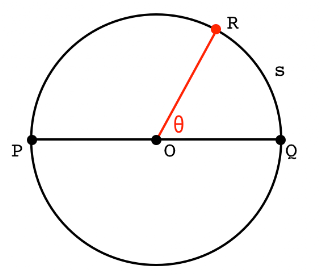In calculus and analytical geometry it is usual to define angles in terms of radians of arc. For a unit circle with radius = 1, the total circumference is 2π, so the arc swept out by the angle θ is in the same ratio to π as the ratio of the angle's measure in degrees to 180°. We say that the angle θ in this figure is equal to the arc it sweeps out on the circumference. And we substitute measures of angles in radians for the ones in degrees:

 180° = π 90° = π/2 60° = π/3 45° = π/4 30° = π/6

Now, think of the same points on the circumference of the circle as forming a triangle. If two points are on a diameter of the circle, the angle at any third point is always a right angle.

To prove: angle PRQ is a right angle.Solution:
Draw the radius OR. Notice that triangles OPR and OQR are both isosceles.
Label the respective base angles a and b.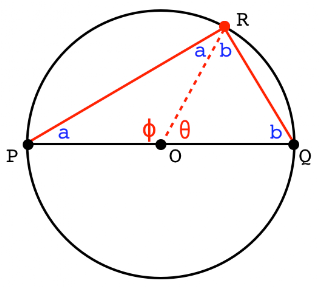2a + φ = π 2b + θ = π 2a + 2b + φ + θ = 2π φ + θ = π

By subtraction (or just by considering that they comprise the angles of triangle PQR):

 2a + 2b = π a + b = π/2

The arc swept out by angles a and b (OPR and OQR on the diameter) clearly add up to π. This suggests that:

 a = 1/2 θ b = 1/2 φ

Solution:

 2a + 2b = π = 2a + φ φ = 2b

Consider the chord PR and draw the tangent at P.The arc between the tangent and the chord equals 2b because it is the same arc as cut off by angle PQR (which is angle b).

Take a chord of the circle, draw the diameter and the tangent.
The same rule applies to both angles: one between the chord and the diameter, and the second between the chord and the tangent. The arc is twice the measure of the angle.

Generalized arc

Having established these basic facts we can do a bit more.
One is to generalize the result for all arcs. The examples so far contain the diameter in some way. Consider the arc swept out by the angle θ in this figure.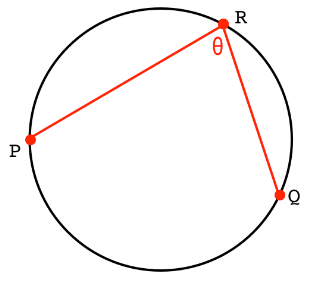We can prove that the measure of the angle θ is equal to the 1/2 the arc swept out between P and Q. For a simple proof, draw the diameter:By our previous work:

 b = 1/2 t a = 1/2 s θ = a + b = 1/2 (s + t)

Intersecting chords.

Given two chords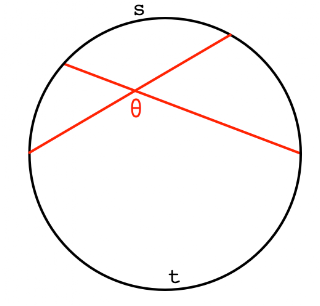To prove:

 θ = 1/2 (s + t)

θ is the average of the two arc lengths.
Solution:
Draw a triangle.a = 1/2 s b = 1/2 t a + b = θ = 1/2(s + t)

Tangent and secant

Rather than having all three points on the circle, one is now outside. We have the same arc swept out by the endpoints (t), but the included angle is now smaller, and there is a new smaller arc of length s.To prove:

 θ = 1/2(t - s)

Solution:
Draw the triangle.By our previous work (and supplementary angles):

 φ = 1/2 s a = 1/2 t

by supplementary angles:

 θ + φ = a θ = 1/2 t - 1/2 s = 1/2(t - s)

Two tangentsDraw a secant line.By our previous work:

 θ1 = 1/2 (t1 - s1) θ2 = 1/2 (t2 - s2)

 θ = 1/2 (t - s)

Two secantsDraw a tangent line.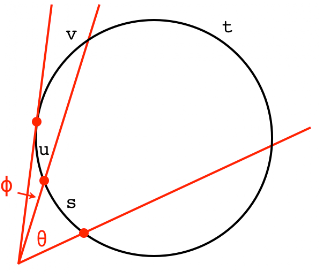By our previous work:

 θ + φ = 1/2(t - s) + 1/2(v - u) φ = 1/2(v - u)

By subtraction:

 θ = 1/2(t - s)

Chord segments

Finally, there is a simple algebraic relationship between chord segments. Draw two chords of the circle and label the lengths of the segments as shown (note: s and t do not refer to arcs any more).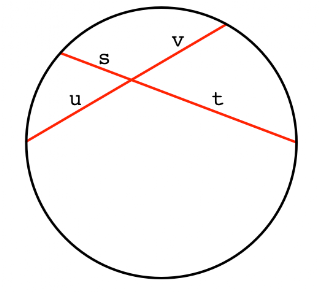To prove:

 st = uv

Solution: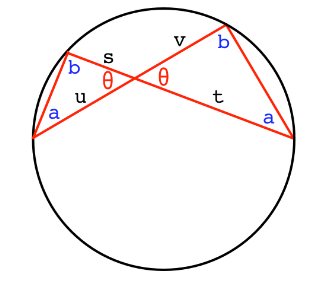Draw the two triangles.
Notice that the two angles labeled a are equal because they sweep out the same arc of the circle, and similarly for the two angles labeled b. By similar triangles:

 s/u = v/t st = uv

Friday, April 1, 2011

Ellipse, again

Michael Starbird is a professor at UT Austin and quite well-known for mathematics teaching (here, here). I've seen (parts of) a bunch of videos he made for The Teaching Company. I like the videos that I got through pretty well, but found them a bit slow-developing. You have to love his energy and enthusiasm though.

Since I was working with ellipses the other day (here), I was reminded of a demonstration he shows in one of the videos, attributed to ____. (Unfortunately, I don't have the videos now, and I've forgotten the name. He's a French mathematician from the early 19th century. If you know, please speak up in comments).

Start with a cylinder, shown in cross section in the graphic (in black).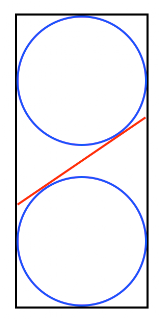The two blue spheres just fit inside the cylinder, but the cylinder is tall enough that the two spheres don't touch, there is some distance between them. We take a cross section of the cylinder (shown in red), and the cross-section is level, so that the two points of the cross-section at each horizontal position are at the same height (the cross-section's extreme bottom and top are at the extreme left and right). The cross-section is an ellipse.

Alternatively, start with an ellipse whose minor axis is the same length as the radius of the cylinder and wedge it tightly inside, then add the spheres so they just touch the ellipse.

We claim that the points where the spheres touch the ellipse are the foci of the ellipse.

That's pretty remarkable. Here is the proof.

In the next figure below, consider any point on the ellipse.On the left, we see the point together with the two foci, and a line drawn from our point to one of the foci. On the right is the cross-section again (foci not shown).

We said that this left focus is the point where the ellipse touches the lower sphere. That is, the line to the focus is a tangent to the sphere. All the tangents to the sphere from this point on the ellipse form a circle. The second tangent of interest is the perpendicular dropped down the surface of the cylinder, shown in the right panel. This line is the same length as line to the focus.

This equality holds for any point on the ellipse.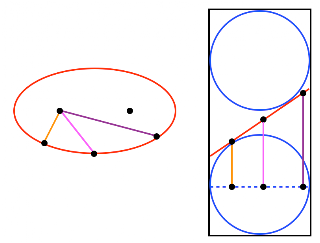Finally, this is true for both spheres. The last figure illustrates that the sum of the perpendicular tangents for any point is a constant. Thus, the points where the spheres touch the ellipse are its foci, because the sum of the distances to any point on the ellipse, which is equal to the sum of the vertical tangents, is a constant.

Simply stunning.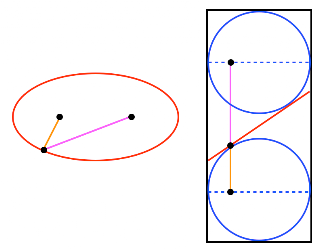Pythagorean TheoremI am a little hesitant to post about a subject (the Pythagorean theorem), which is so well-known and covered so thoroughly on the web. Bronowski calls this relationship the "the most important single theorem in the whole of mathematics." (Ascent of Man: Ch. 4, Music of the Spheres). And I find these geometric proofs really wonderful to behold.

The graphic shows a right triangle and the squares of the sides in what is sometimes called the "Bride's Chair" form.

Let's start with the end rather than the beginning, and consider the last step. Drop an altitude in the right triangle as indicated by the dotted line. The argument will demonstrate that the two shaded areas in the figure (plum and red) are equal. That is the crux of the proof.

Then, by considering the symmetry of the figure, it is easy to see that the whole area of the red square is equal to the sum of the other two squares.

Now go back one step to the middle of the proof. The insight of the mathematician (who is really unknown) was to consider two special triangles as shown in the second figure (ADE on the left and ABG on the right).Start with ADE. We take AE as the base of the triangle. Because the vertex D is on a line extended through FG, the height of the triangle is equal to EF, and therefore its area is one-half the area of the plum-colored square in the first figure.

Now look at ABG in the right panel. The altitude we dropped crosses AD at point H (marked as a red dot), and the shaded area we want is that part of the red square to the left of the dotted line, the area of rectangle ABIH. Take AB as the base of the triangle ABG. Then its height is equal to AH, and therefore its area is one-half the area of ABHI.

The first part of the proof is to show that these two carefully chosen triangles, ADE and ABG, are congruent (equal). The reason is SAS (side-angle-side). The side part is obvious. To get the angle, notice that ABG is just ADE rotated clockwise 90 degrees. Or, let the angle GAD = θ. Then, angle EAD = 90° + θ, but angle GAB = θ + 90°, which is the same.

This first proof illustrates a point about mathematical and scientific insight---its stepwise or incremental nature. We reach the summit by finding the crucial intermediate hand- and toe-holds.

There are many, many proofs of the Pythagorean theorem (e.g. here).

My absolute favorites are the geometric ones (#3 and #9), which are so simple that they really require no words. Here is #9, redrawn: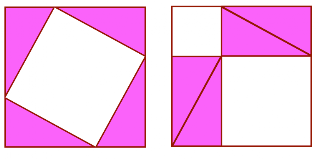And finally, we can do it algebraically, although it feels a bit like cheating. Using the figure above, label the sides of the right triangle as a, b and the hypotenuse h. Then the sides of the large square on the left are a + b and its area is:

 (a + b)2 = a2 + 2ab + b2

while the individual pieces are:

 = 4(1/2)ab + h2

Cancel and obtain:

 a2 + b2 = h2

For a second method, drop the altitude h in the right triangle below, to divide the hypotenuse c into d + e. Notice that the two smaller triangles are similar to the original large one.By similar triangles:

 c/a = a/dcd = a2c/b = b/ece = b2a2 + b2 = cd + ce = c(d + e) = c2